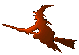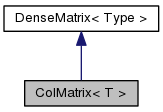Hex  1.0 Hydrogen-electron collision solver
ColMatrix< T > Class Template Reference

Dense (column-oriented) matrix. More...

#include <matrix.h>

Inheritance diagram for ColMatrix< T >:[legend]
Collaboration diagram for ColMatrix< T >:[legend]

## Public Member Functions

ColMatrix ()

ColMatrix (int size)

ColMatrix (int rows, int cols)

ColMatrix (int rows, int cols, const ArrayView< Type > data)

ColMatrix (DenseMatrix< Type > const &M)

template<class Function >
void populate (Function f)
Populator. More...

RowMatrix< Type > T () const
Matrix transpose. More...

ArrayView< Type > col (int i)
Matrix column. More...

const ArrayView< Type > col (int i) const

Type operator() (int i, int j) const
Element access. More...

Type & operator() (int i, int j)Public Member Functions inherited from DenseMatrix< Type >
DenseMatrix ()

DenseMatrix (int rows, int cols)

DenseMatrix (int rows, int cols, const ArrayView< Type > data)

ArrayView< Type > data ()

const ArrayView< Type > data () const

size_t size () const

int cols () const

int rows () const

Type * begin ()

Typeconst * begin () const

## Detailed Description

### template<class T> class ColMatrix< T >

This class represents a generally non-symmetric dense matrix. It is an encapsulation of the NumberArray class with some operators bundled to it.

## Constructor & Destructor Documentation

template<class T>
 ColMatrix< T >::ColMatrix ( )
inline
template<class T>
 ColMatrix< T >::ColMatrix ( int size )
inline
template<class T>
 ColMatrix< T >::ColMatrix ( int rows, int cols )
inline
template<class T>
 ColMatrix< T >::ColMatrix ( int rows, int cols, const ArrayView< Type > data )
inline
template<class T>
 ColMatrix< T >::ColMatrix ( DenseMatrix< Type > const & M )
inlineexplicit

## Member Function Documentation

template<class T>
 ArrayView ColMatrix< T >::col ( int i )
inline

Return shallow copy of the chosen matrix column.

template<class T>
 const ArrayView ColMatrix< T >::col ( int i ) const
inline
template<class T>
 Type ColMatrix< T >::operator() ( int i, int j ) const
inline
template<class T>
 Type& ColMatrix< T >::operator() ( int i, int j )
inline
template<class T>
template<class Function >
 void ColMatrix< T >::populate ( Function f )
inline

This method accepts a function object that is called for every matrix element.

template<class T>
 RowMatrix ColMatrix< T >::T ( ) const
inline

Return transposed matrix. The result is of the type RowMatrix, so that no reordering of the entries is necessary.

The documentation for this class was generated from the following file: